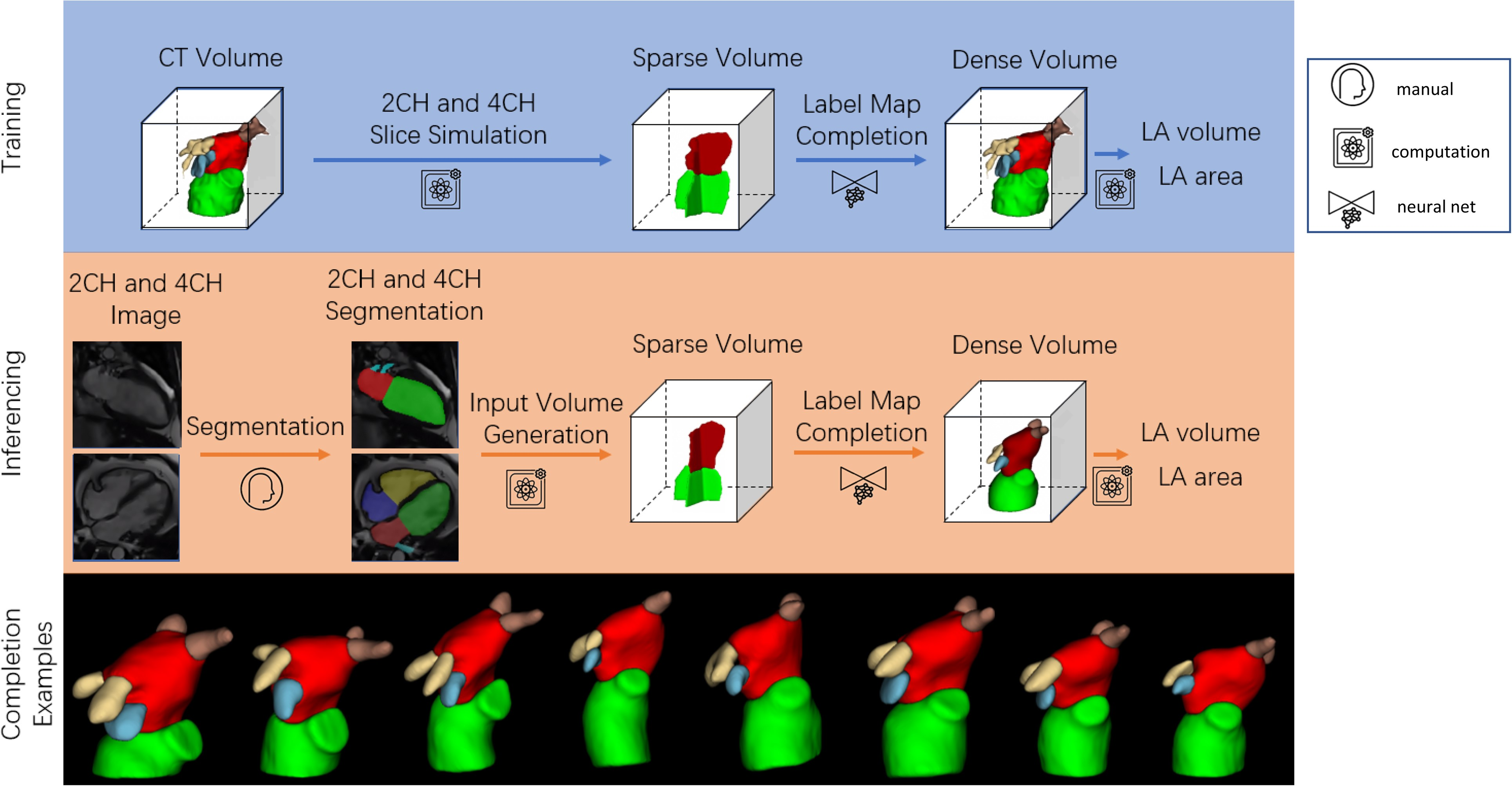## LA MR reconstruction

Left atrial volume is commonly estimated using the bi-plane area-length method from two-chamber (2CH) and four-chamber (4CH) long axes views. However, this can be inaccurate due to a violation of geometric assumptions. We aimed to develop a deep learning neural network to infer 3D left atrial shape, volume and surface area from 2CH and 4CH views.

A 3D UNet was trained and tested using 2CH and 4CH segmentations generated from 3D coronary computed tomography angiography (CCTA) segmentations (n = 1700, with 1400/100/200 cases for training/validating/testing). An independent test dataset from another institution was also evaluated, using cardiac magnetic resonance (CMR) 2CH and 4CH segmentations as input and 3D CCTA segmentations as the ground truth (n = 20). For the 200 test cases generated from CCTA, the network achieved a mean Dice score value of 93.7%, showing excellent 3D shape reconstruction from two views compared with the 3D segmentation Dice of 97.4%. The network also showed significantly lower mean absolute error values of 3.5 mL/4.9 cm2 for LA volume/surface area respectively compared to the area-length method errors of 13.0 mL/34.1 cm2 respectively (P < 0.05 for both). For the independent CMR test set, the network achieved accurate 3D shape estimation (mean Dice score value of 87.4%), and a mean absolute error values of 6.0 mL/5.7 cm2 for left atrial volume/surface area respectively, significantly less than the area-length method errors of 14.2 mL/19.3 cm2 respectively (P < 0.05 for both).

Compared to the bi-plane area-length method, the network showed higher accuracy and robustness for both volume and surface area.

You can read more details about the network in Deep learning estimation of three-dimensional left atrial shape from two-chamber and four-chamber cardiac long axis views. In the reconstruction kit below you can find the MR inference code and the trained model.